edHelper subscribers - Create a new printable

Sample edHelper.com - Visual Perceptual Skills Worksheet

 Name _____________________________Date ___________________ (Answer ID # 0887688)
Pick the choice that is a correct statement.
1.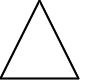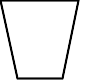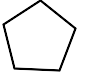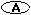the pentagon is to the left of the triangle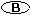the triangle is to the right of the pentagon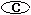the triangle is between the pentagon and the trapezoid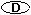the triangle is to the left of the pentagon
2.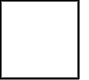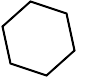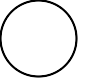the hexagon is to the left of the circlethe square is to the right of the hexagonthe hexagon is to the right of the circlethe hexagon is third
3.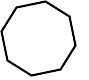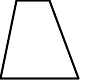the octagon is to the right of the trapezoidthe trapezoid is secondthe trapezoid is to the right of the squarethe octagon is to the right of the square
4.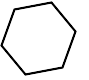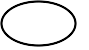the oval is to the left of the hexagonthe hexagon is thirdthe hexagon is to the left of the ovalthe hexagon is between the triangle and the oval
5.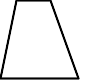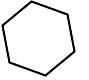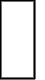the hexagon is firstthe trapezoid is to the right of the hexagonthe rectangle is to the left of the hexagonthe hexagon is between the trapezoid and the rectangle
6.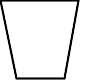the rectangle is firstthe rectangle is to the left of the trapezoidthe triangle is to the right of the rectanglethe rectangle is third